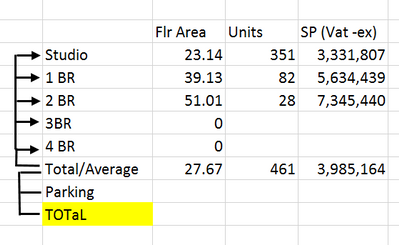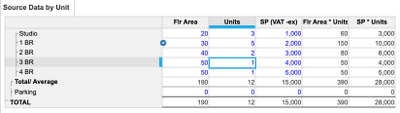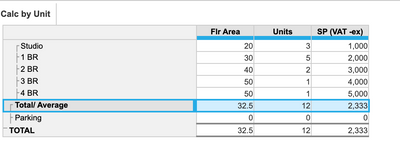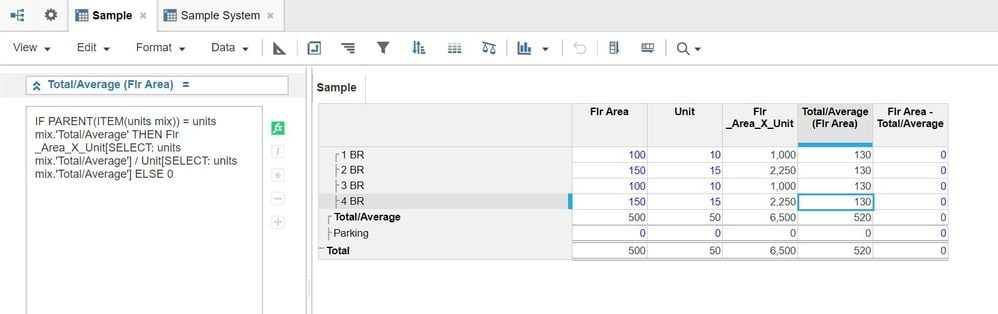# Circular Reference Issue in ComputationHi All,

I would like to ask for some insights with a Circular Reference Issue. Here are the details

So we have a dimension Unit Mix, where the TOTAL is the Top Level and Parking and Total/ Average is the child. And under Total/Average are child member 1 BR, 2 BR, 3 BR, 4 BR. ( I hope i was able to illustrate the dimension properly).

The clients requirement is to calculate the Total/Average of the 4 Unit Mix without the Parking as they separate the calculation for this. What we are trying to calculate is the Total/Average for Flr Area and SP (Vat-ex).

Formula

Total/Average (Flr Area) = (Flr Area (Studio)*Unit(Studio)) + (Flr Area (1 BR) *Unit (1BR))+ (Flr Area(2BR)*Unit(2BR)) + (Flr Area (3BR))*Unit(3BR)) + Flr Area(4BR) * Unit *4BR)) /Unit(Total/Average

Total/Average (SP(VAT -ex))=(SP VAT-ex (Studio)*Unit(Studio)) + (SP VAT-ex (1 BR) *Unit (1BR))+ (SP VAT-ex(2BR)*Unit(2BR)) + (SP VAT-ex (3BR))*Unit(3BR)) + SP VAT-ex (4BR) * Unit *4BR)) /Unit(Total/Average

I tried to use Flr Area[select:Studio]*Units[select:Studio] and I got the Circular reference. I even tried to create a duplicate version of the module and refer it to that (since it worked in the past) but still getting the same errorAny ideas? Any help would be appreaciated

Regards,

Tagged:

•Hi @Jsdeloria21,

Can you clarify my doubts so that i will do analyse well.

1. i assume Flr Area, units, SP(VAT -ex) are line items and Unit Mix is a dimension of a module.

2. Total/Average (Flr Area) = (Flr Area (Studio)*Unit(Studio)) + (Flr Area (1 BR) *Unit (1BR))+ (Flr Area(2BR)*Unit(2BR)) + (Flr Area (3BR))*Unit(3BR)) + Flr Area(4BR) * Unit *4BR)) /Unit(Total/Average

is it a formula written on Flr Area line item...?

3. Total/Average (SP(VAT -ex))=(SP VAT-ex (Studio)*Unit(Studio)) + (SP VAT-ex (1 BR) *Unit (1BR))+ (SP VAT-ex(2BR)*Unit(2BR)) + (SP VAT-ex (3BR))*Unit(3BR)) + SP VAT-ex (4BR) * Unit *4BR)) /Unit(Total/Average

is it a formula written on SP(VAT -ex) line item...?

4. I tried to use Flr Area[select:Studio]*Units[select:Studio] and I got the Circular reference

in which module are you writing this formula..?

and what is the line item name...?

Thanks,

•Hi @Jsdeloria21 ,

Assuming it is a ragged hierarchy (although personally do not prefer it), the following solution can work:

1. Create two line items in your source module

• Flr Area * Units
• SP * Units

Plug in the formula according to the attached blueprint.2. Create another module with the "Unit Mix" dimension and the line items that you need. Use the formulas in the second module to calculate the weighted average for the top level. In this module, the summary method needs to be set as "Formula"There might be scope of future optimization. Let me know if this works.

•@Jsdeloria21  If it was a hierarchical list, then I think you would have been able to solve it pretty straightforward. Anyways, have a look at the solution in case of a ragged hierarchy.

•Does all the items in the list need to be part of one list (i.e. ragged hierarchy)?

•1. i assume Flr Area, units, SP(VAT -ex) are line items and Unit Mix is a dimension of a module.

- Yes.

2. Total/Average (Flr Area) = (Flr Area (Studio)*Unit(Studio)) + (Flr Area (1 BR) *Unit (1BR))+ (Flr Area(2BR)*Unit(2BR)) + (Flr Area (3BR))*Unit(3BR)) + Flr Area(4BR) * Unit *4BR)) /Unit(Total/Average

- is it a formula written on Flr Area line item...? - It is written on the Total/Average - Flr Area column.

3. Total/Average (SP(VAT -ex))=(SP VAT-ex (Studio)*Unit(Studio)) + (SP VAT-ex (1 BR) *Unit (1BR))+ (SP VAT-ex(2BR)*Unit(2BR)) + (SP VAT-ex (3BR))*Unit(3BR)) + SP VAT-ex (4BR) * Unit *4BR)) /Unit(Total/Average

is it a formula written on SP(VAT -ex) line item...?

- It is written on the Total/Average  SP (Vat - ex) Column

4. I tried to use Flr Area[select:Studio]*Units[select:Studio] and I got the Circular reference

in which module are you writing this formula..?

- on the same module, Under Flr Area - Total/Average. I did a IF item(Unit Mix) = Unit Mix.Total Average then Flr Area[select:Studio]*Units[select:Studio]

and what is the line item name...?

•Hi Anikdas,

Base on the template of the client they have Total/Average and a Total on the table. But if there is a way that you can compute it separate I am open to that option as well.

Thanks.

•Hi @Jsdeloria21,

Try this if my approach works.

I am using select statement here because i am not 100% clear on your complete requirement and moreover you want to write a different logic for PARKING, that's why